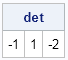One of my favorite features of SAS/IML 12.1 (released with 9.3m2) is that the USE and CLOSE statements support reading data set names that are specified in a SAS/IML matrix. The IMLPlus language in SAS/IML Studio has supported this syntax since the early 2000s, so I am pleased that this feature has finally made its way into PROC IML.

It is very convenient to be able to read multiple SAS data sets in a loop. For example, suppose that you want to read the contents of the following SAS data sets into SAS/IML matrices:

```data A; /* create square matrix */ x=1; y=2; output; x=2; y=3; output; run; data B; set A; x = x + 2; run; data C; set A; y = y + 1; run;```

If you love typing, you can read these data sets by explicitly writing three USE, READ, and CLOSE statements:

```proc iml; use A; read all var _NUM_ into A; close A; use B; read all var _NUM_ into B; close B; use C; read all var _NUM_ into C; close C;```

But what if there are many data sets to process? Or what if the names of the data sets are not known until run time? (For example, the names are stored in a file.) If you are a macro programmer, you might try to automate this process by writing a macro, as was described recently on StackOverflow. Personally, I prefer to avoid using macros in SAS/IML programs when I have the option.

An alternative is to write a program that reads data sets whose names are specified in an array. The USE and CLOSE statements in SAS/IML 12.1 support a simple way to read data set names when the names are stored in a SAS/IML matrix. For example, the following statements read a data set in the Sashelp library:

```ds = "Sashelp.Class"; use (ds); read all var _NUM_ into X; close (ds);```

The use of parentheses on the USE and CLOSE statements is required. Without the parentheses, PROC IML will look for a data set named "DS", which does not exist. The parentheses tell PROC IML to "look inside" (or dereference) the ds variable.

You can loop over the data sets in the Work library to perform matrix operations on each data set. The following example computes the matrix determinant of the matrices that are stored in the A, B, and C data sets:

```dsNames = {A B C}; /* specify names of data sets */ det = j(1, ncol(dsNames)); /* allocate matrix for results */ do i = 1 to ncol(dsNames); use (dsNames[i]); /* open work.A, then work.B, and so on */ read all var _NUM_ into X; /* read data into X */ det[i] = det(X); /* do some matrix computation */ close (dsNames[i]); end; print det;```The previous code reads the data for each data set into the matrix X. The value of the determinant of the matrix is calculated and saved in the det vector, which is printed after all data sets are processed.

The example code performs the same computation on each data set. In my next article, I will show how you can read multiple data sets into SAS/IML matrices and assign each matrix a different name. This alternate technique is useful when you want to read matrices from several data set and have all of the matrices exist simultaneously.

Share